# NCERT Solutions for Class 9 Maths Chapter 10 Exercise 10.2 – Circles

Download NCERT Solutions for Class 9 Maths Chapter 10 Exercise 10.2 – Circles. This Exercise contains 2 questions, for which detailed answers have been provided in this note. In case you are looking at studying the remaining Exercise for Class 9 for Maths NCERT solutions for Chapter 10 or other Chapters, you can click the link at the end of this Note.

### NCERT Solutions for Class 9 Maths Chapter 10 Exercise 10.2 – Circles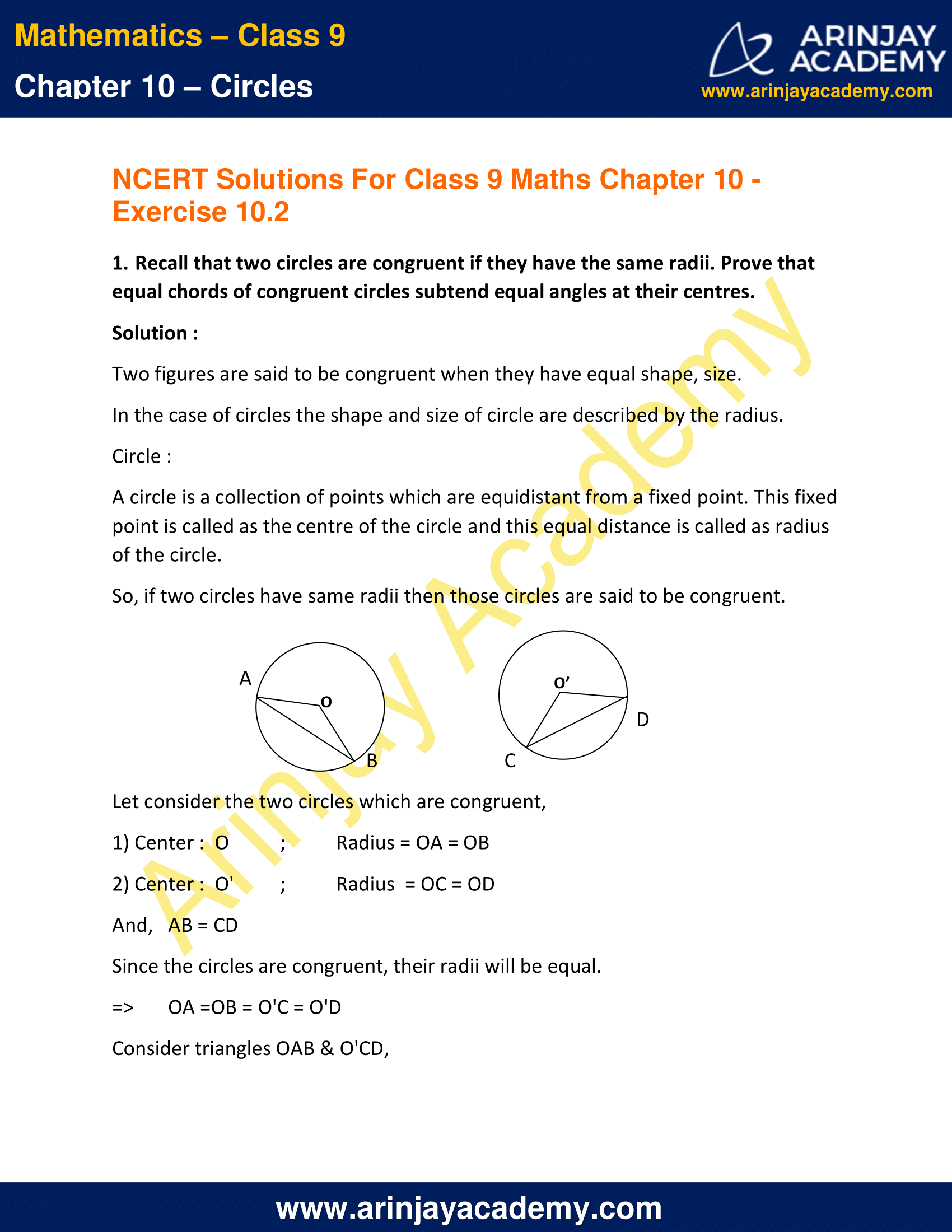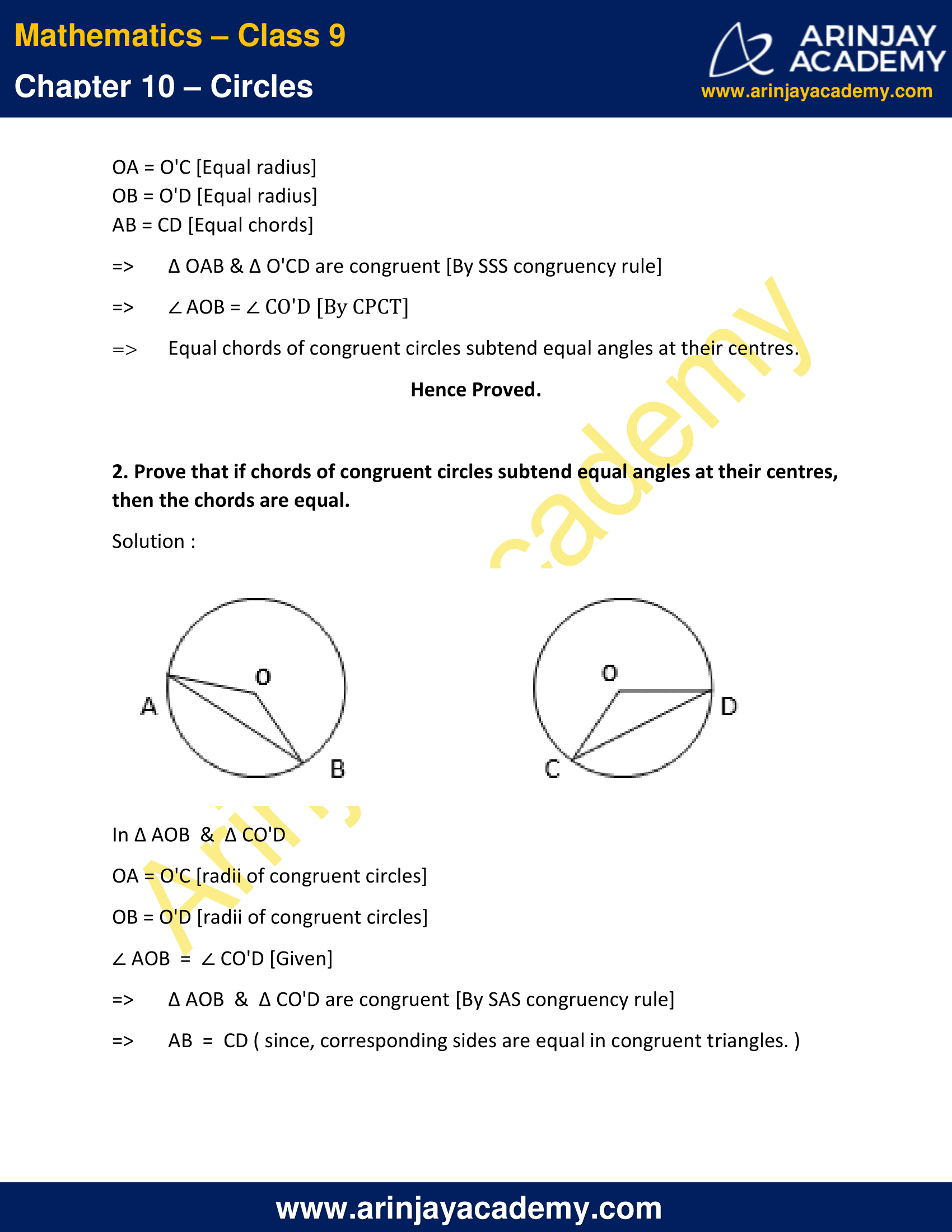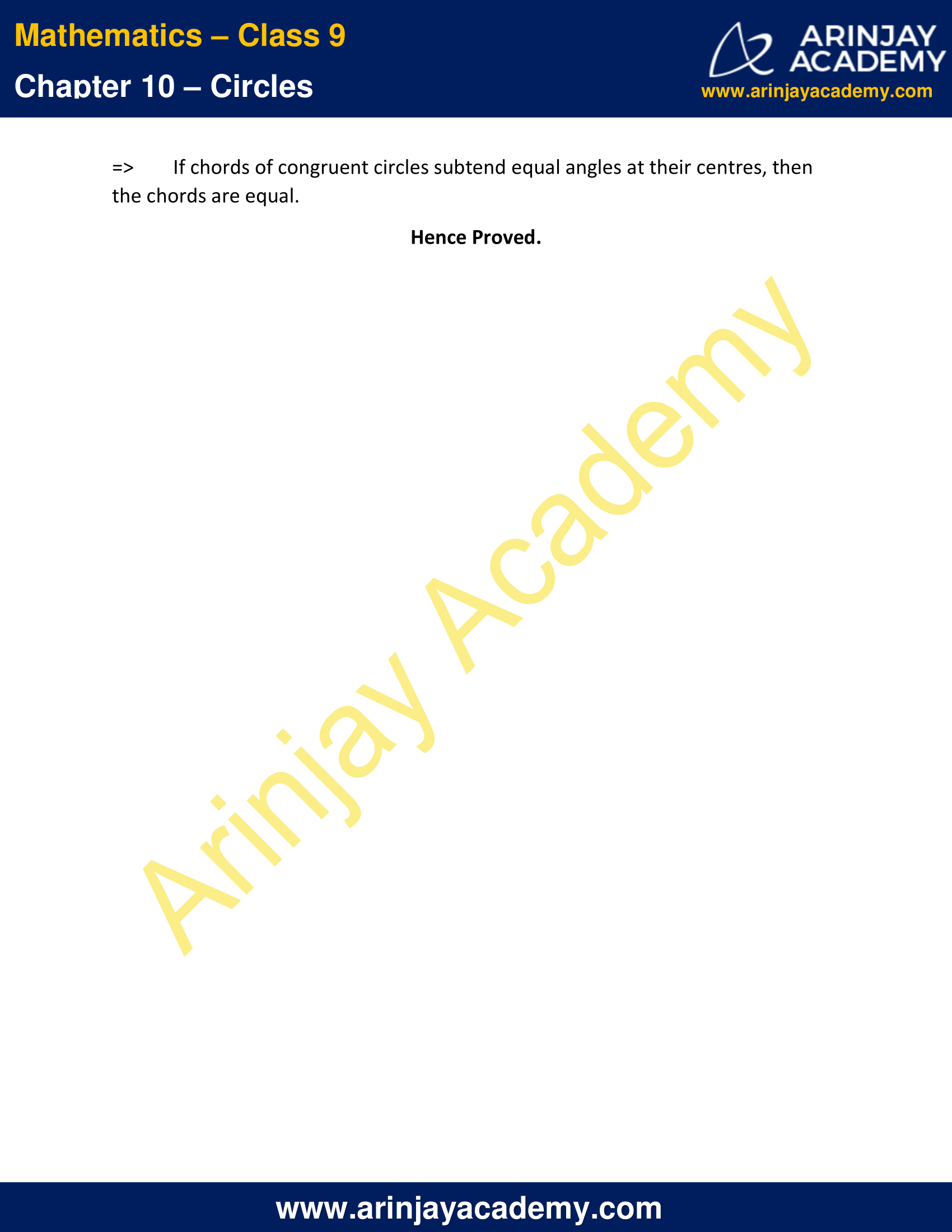NCERT Solutions for Class 9 Maths Chapter 10 Exercise 10.2 – Circles

1. Recall that two circles are congruent if they have the same radii. Prove that equal chords of congruent circles subtend equal angles at their centres.

Solution :

Two figures are said to be congruent when they have equal shape, size.
In the case of circles the shape and size of circle are described by the radius.

Circle :

A circle is a collection of points which are equidistant from a fixed point. This fixed point is called as the centre of the circle and this equal distance is called as radius of the circle.

So, if two circles have same radii then those circles are said to be congruent.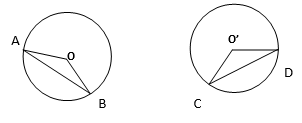Let consider the two circles which are congruent,

1) Center :  O ; Radius = OA = OB
2) Center :  O’ ; Radius  = OC = OD
And,   AB = CD
Since the circles are congruent, their radii will be equal.
=> OA =OB = O’C = O’D
Consider triangles OAB & O’CD,
OA = O’C [Equal radius]
OB = O’D [Equal radius]
AB = CD [Equal chords]
=>  ∆ OAB & ∆ O’CD are congruent [By SSS congruency rule]
=>  ∠ AOB = ∠ CO’D [By CPCT]
=>  Equal chords of congruent circles subtend equal angles at their centres.
Hence Proved.

2. Prove that if chords of congruent circles subtend equal angles at their centres, then the chords are equal.

Solution :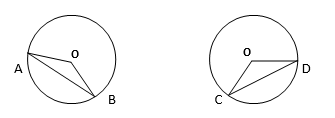In ∆ AOB  &  ∆ CO’D
OA = O’C [radii of congruent circles]
OB = O’D [radii of congruent circles]
∠ AOB  =  ∠ CO’D [Given]
=> ∆ AOB  &  ∆ CO’D are congruent [By SAS congruency rule]
=>  AB  =  CD ( since, corresponding sides are equal in congruent triangles. )
=> If chords of congruent circles subtend equal angles at their centres, then the chords are equal.

Hence Proved.

NCERT Solutions for Class 9 Maths Chapter 10 Exercise 10.2 – Circles, has been designed by the NCERT to test the knowledge of the student on the topic – Angle Subtended by a Chord at a Point

The next Exercise for NCERT Solutions for Class 9 Maths Chapter 10 Exercise 10.3 – Circles can be accessed by clicking here.

Download NCERT Solutions for Class 9 Maths Chapter 10 Exercise 10.2 – Circles